# Dan's Blog

Musings on code, and other assorted sundries

# Propagation of Real-Time Terrain Deformations in Chunked LOD Style Terrains

Real-time deformation of terrain is a necessary component of many styles of games. Strategy games that require structure placement need this ability in order to reconcile differences in height. Other styles of game might need this for terrain destruction as the result of, say, a cluster bomb. Packaged level editors certainly need this ability.

The necessity of real-time deformation means that the choice of terrain systems is typically limited. At its most basic, a simple plane (or tiles) with no varying level of detail is the obvious choice. Deformation of vertices in this case requires no special treatment other than assigning a new Y coordinate (assuming Y is “up”, and that your simulation has a traditional sense of gravity), and some normal recalculation. The obvious drawback of this though is that you are limited in either the size of your terrain, detail, and/or camera angles you can support before the frame rate reaches an unacceptably low level.

This limitation is not necessary though. In this article, I’ll be demonstrating my method for deforming chunked LOD terrain via index scaling. By index scaling, I mean quickly determining the index of a vertex in a child chunk that shares the same space as a vertex in its parent. In addition, we narrow the search area to further increase the speed of the index search.

A few things before we begin. All code samples will be provided in C#, and make use of the Unity3D library. In addition, we will be using a coordinate system where Y is up, and Z is forward (as is the case in Unity). To avoid complicating things, we will discuss deformations happening in a single chunk. In reality, changes will very often happen across chunk boundaries (add therefore affect neighboring chunks). This leads to a need to reconcile the edges; both the vertex positions and the normals. This is out of scope of this article though, and will be avoided. Just remember that the methodology is the same in this case; you simply would repeat these steps for each affected chunk (and then reconcile the edges).

Another thing we need to ensure is that we are using chunks with well ordered indices. All chunks in the terrain, of all levels of detail, should use the same exact index ordering (and same number of vertices). In this article, our chunks will have its forward-leftmost corner as index 0, and its closest-rightmost corner index as vertex count – 1, like so: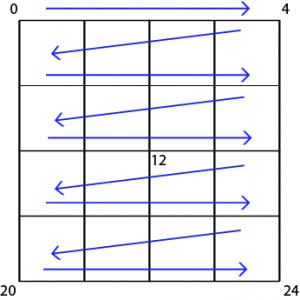The first thing we do is to apply our vertex changes (position and normal) to the highest LOD chunk (whether it is visible on screen or not). For the purpose of this example, let’s say we are deforming a portion of the chunk to create a flat mesa; that is to say, we are moving the vertices in a radius to a per-determined Y value. Here is what a function to do such a thing might look like:

``````public void CreateMesa(float height, float radius, Vector3 origin) {
// zero out the y coordinate of the origin so that we can
// perform a distance check on a single plane
origin.y = 0;

Vector3[] vertices = mesh.vertices;
for(int i = 0; i < vertices.length; i++) {
Vector3 v0 = vertices[i];

// zero out the y coordinate of the vertex
// we are testing so that the distance is
// measured on a single plane, to match our change
// to origin.
v0.y = 0;

vertices[i].y = height;
}
}

mesh.vertices = vertices;
mesh.RecalculateNormals();
}``````

Let's talk about this method for a bit. It is fairly straight forward; we scan each vertex of the chunk to see if it is within the radius of the center of the mesa, and if it is, we alter the height. We zero out the y values for testing because we want to affect an area in the X and Z planes. In other words, we want to test the area as if it were the radius of a cylinder, and not the radius of a sphere.

Now that we have a deformation to propagate, we need to recursively move up the tree of chunks until we reach the root level chunk. How you choose to store your chunked LOD is unimportant for this. What is important is that a way exists to find the chunk's parent, grandparent, great-grandparent, and all ancestors right up to the lowest LOD.

For each LOD, we need to find the vertices that exist in both the parent, and the child. While each chunk has the same index order, and vertex count; the size is twice that of its direct descendant. What this means is that every other vertex in the child has no corresponding vertex in the parent. To complicate things further, each chunk has four children. As such, each index does not always match up in the same position of a vertex with the same index as the parent (in fact, it only does four times):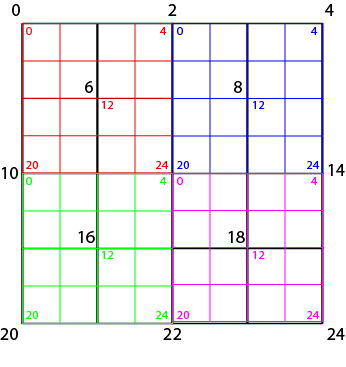The naive approach would be to scan each vertex to see if it shares the same X and Z coordinate of its child; but this is terribly slow, as we need to scan each vertex of both the parent and child, as well as perform a distance calculation for each vertex; which means a run-time of O(n2). We can instead rely on some simple properties regarding the relationship between a child's vertices, and its parent:

1. Only vertices which are in the same quadrant of the parent chunk that the child chunk resides in can have matching vertices
2. Only vertices with even numbered indices in the child can exist in both parent and child
3. Only even numbered "rows" and "columns" of vertices can have vertices that exist in both parent and child
4. Our chunks contain an even number of faces, and an odd number of verticesThe first rule is easy enough to follow; it completely depends on how you store your chunks, but that is trivial. Simply being able to know if the child is in the top-left, top-right, bottom-left, or bottom-right quadrants is what is important. For our purposes, let's assume our quadtree keeps track of chunks like so:

``````public class ChunkNode<T> {
T chunk;
ChunkNode   parent
ChunkNode[] children;

TopLeft     = 0,
TopRight    = 1,
BottomLeft  = 2,
BottomRight = 3
}

public ChunkNode(T chunk, ChunkNode parent) {
this.chunk = chunk;
this.parent = parent;
children = new ChunkNode;
}

}
}``````

This is a simple representation of what a node in a quadtree might look like if keeping track of the quadrant position of the chunk relative to its parent.

The second and third rules, while helpful to know for understanding the relationship between a parent chunk and a child chunk, can actually be ignored. The reason for this is that we will be iterating over parents using a formula to determine the index of the child vertex for each vertex we are testing in the parent.

The fourth rule is what allows us to easily find the index in the child corresponding to the index in the parent. In order to find the index in the child of the vertex we are examining, we will be using this simple formula*: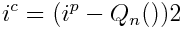In this equation, ic represents the child vertex index; ip represents the parent vertex index. Qn() is one of the following four functions that calculate an offset relative to the quadrant the child exists in: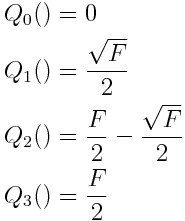in other words:

``````int childIndex = (parentIndex - quadrantOffset) * 2;

// or the faster equivalent:
int childIndex = (parentIndex - quadrantOffset) << 1;``````

*This assumes that each of your chunk's LODs are a single mesh. If your chunks' LODs are all in the same mesh, and you are simply adjusting the index buffer to change levels; you would need to add an additional offset based on the LOD (using the example image above: 0, 25, 50, etc). Each LOD should still be arranged as described above though

What this does is first subtract an offset value determined by the quadrant the child exists in relative to the parent. Then, we simply multiply by two to "scale the index up". The resulting number is the index in the child for the vertex in the same position as the parent. Fast and simple!

The quadrant offset functions are fairly simple as well. F denotes the quad count of the chunk. Since all chunks will share the same vertex and quad count, these values can be pre-calculated. We need the offsets because the vertices are stored in a single dimension; we need to apply these offsets in order to find the index in the appropriate chunk.

Here is a code snippet illustrating this:

``````int quadCount;

public void Init() {
// in our example, we'll use 4x4 quad chunks

// pre-calculate the offsets
0,
};
}

}``````

Now that we have a way to map a parent index to a child index in the same position, we need a way to restrict the scan to just the vertices in the parent that are in the same quadrant as the child. Doing this is trivial; it is simply a matter of using correct index offsets. Consider the following image: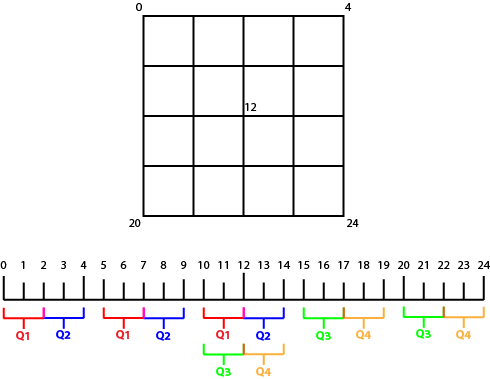This image shows the vertex indices for a chunk of 4x4 quads (5x5 vertices) for a total of 25 vertices. The vertices belonging to each quadrant are in an identifiable pattern that we can use to restrict the scan to the affected quadrant. Each range of indices is half the vertex count of a side (or the square root of the total vertices), rounded up to the next whole number. Since we are working with 0 based indices though, we actually will floor the results. To put it concisely: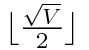Where V is the total vertex count. This formula also determines the number of indices we need to skip in order to get to the next "row" of vertices. Process 3, skip 2, process 3, skip 2, process 3.

A variant of this formula is used to determine how many times we need to loop over a set to get to the end of the quadrant's vertices; we simply round up. In this example, we have 3 vertices per "row", and need to loop 3 times to process each "column"

There is also another pattern evident in the example: where to begin the scan. For the top-left quadrant, this is simply index 0. For all the others however, we need to start somewhere further up. This depends on the quadrant, much like when we convert a parent index do a child index in the same space. In fact, the equations are nearly exactly the same: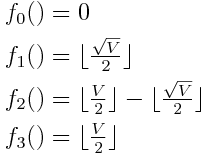The following code snippet illustrates how we can apply this information for scanning only the subset of vertices we care about:

``````// Find the start index offset for the beginning of the scan
// based on the quadrant our child lives in.
int startIndex = 0;
startIndex = Math.Floor(Math.Sqrt(vertexCount) / 2);
break;
startIndex = Math.Floor(vertexCount / 2) - Math.Floor(Math.Sqrt(vertexCount) / 2);
break;
startIndex = Math.Floor(vertexCount / 2);
break;
}

// How many indices are scanned per row?
int indicesScanCount = Math.Ceil(Math.Sqrt(vertexCount) / 2);

// Loop over each "row" and "col" of indices. For each
// row, skip ahead to start the next iteration at the
// correct index.
int index = startIndex;
for(int row = 0; row < indicesScanCount, row++) {
for(int col = 0; col < indicesScanCount, col++) {
index += col;
}
index += indicesScanCount;
}``````

This code can easily be made more efficient by caching the calculations for `indicesScanCount`, as well as all of the index offsets based on quadrant.

Now we have everything we need to put this all together:

``````public class Chunk {
// stores the geometry of our chunk;
public Mesh mesh;
}

public class ChunkNode<T> {
public T chunk;
public ChunkNode<T>  parent
public ChunkNode<T>[] children;

TopLeft     = 0,
TopRight    = 1,
BottomLeft  = 2,
BottomRight = 3
}

this.chunk = chunk;
this.parent = parent;
children = new ChunkNode;
}

}
}

public class TerrainDeformer {
int vertexCount;
int vertexCountSquared;

int indicesScanCount;

// vertex count is always "rows" + "columns" + 1
vertexCountSquared = (int)Math.Sqrt(vertexCount);

// pre-calculate the scaling offsets
0,
};

// pre-calculate the scanning offsets
0,
Math.Floor(vertexCountSquared / 2),
Math.Floor(vertexCount / 2) - Math.Floor(vertexCountSquared / 2),
Math.Floor(vertexCount / 2),
};

// how many indices do we scan per "row", and for how many "cols"
int indicesScanCount = Math.Ceil(Math.Sqrt(vertexCount) / 2);
}

}

public void PropagateDeformationToAncestors(ChunkNode<Chunk> chunkNode) {
// seed our scan
ChunkNode<Chunk> parent = chunkNode.parent;
ChunkNode<Chunk> child = chunkNode;

while(parent != null) {
// get the chunks' mesh
Mesh parentMesh = parent.chunk.mesh;
Mesh childMesh = child.chunk.mesh;

// get the chunks' vertices
parentVertices = parentMesh.vertices;
childVertices = childMesh.vertices;

// Start from the correct offset. The quadrants can
// be different as we move up the tree
for(int row = 0; row < indicesScanCount, row++) {
for(int col = 0; col < indicesScanCount, col++) {
// current parent index
index += col;

// find the related child index
int childIndex = ScaleIndexToChild(index);

parentVertices[index].y = childVertices[childIndex].y;
}

// skip some indices
index += indicesScanCount;
}

// bake the mesh
parentMesh.vertices = parentVertices;
parentMesh.recalculateNormals();
parent.chunk.mesh = parentMesh;

// move up to the next parent
child = parent;
parent = child.parent;
}
}
}``````

The above example is very basic, and is intended to demonstrate the bare minimum required to propagate the deformation of a single chunk. In a real world scenario, you would deform each relevant highest LOD chunk, create a `TerrainDeformer` object, and call `PropagateDeformationToAncestors()` on each chunk you modified. You could also further optimize it by making some of those calculations static, so that they only need to be calculated once for the duration of the program.

Here are some other optimizations that could be made to make this even more efficient:

• Leverage the fact that as we go to lower and lower levels of detail, deforms will happen in a smaller and smaller area relative to the chunk. The above equations could be modified to take this into account, reducing the scanning time even further.
• Keep track of indices that have been altered, so that deforms of neighboring chunks can potentially skip indices that are shared by neighbors that share the same parent (in our example image above, indices 2, 7, 10, 12, 13, 14, and 15 could potentially be skipped)

So as you can see, it is possible to efficiently propagate deforms up the chunk tree, all the way to the root chunk. This is not without its drawbacks of course. As the deform gets to lower and lower levels of detail, the resolution suffers. With a very small deformation, it is possible for a deformation to disappear entirely once it reaches a low enough LOD. This might not be an issue if your deformations are very large and uniform (like hill creation), or small and not noticeable from a distance (a minor adjustment to bring land level to a building's foundation). There are a number of ways this can be solved, but that is out of scope of this article.